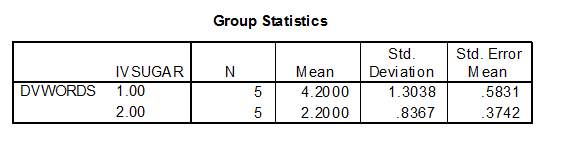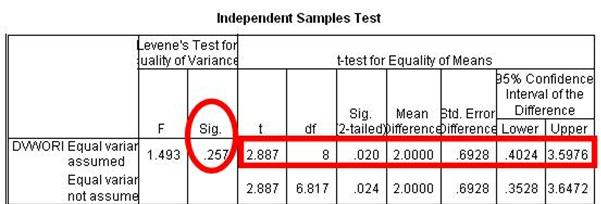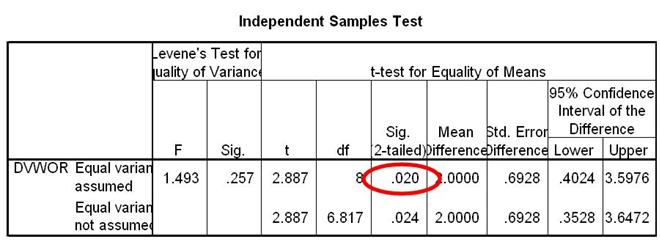How do I interpret data in SPSS for an independent samples T-test?

Home > How do I interpret data in SPSS for an independent samples T-test?## Group Statistics Box

Take a look at the first box in your output file called Group Statistics. In the first column, you will see the number 1 and the number 2 under the word IVSUGAR. These are the numbers that we chose to represent our two IV conditions, sugar (1) and no sugar (2). You can find out some descriptive statistics about each condition by reading across each row in this box. You can also see the number of participants per condition.## Example

In the Group Statistics box, the mean for condition 1 (sugar) is 4.20. The mean for condition 2 (no sugar) is 2.20. The standard deviation for condition 1 is 1.30 and for condition 2, 0.84. The number of participants in each condition (N) is 5.

## Why look at Group Statistics

Some students wonder why we look at this box. We are doing a T-test and this box does not tell us the results for that test. We look at the box because it can give us some important and relevant information.

## Example

We can see how many data points were entered for each condition. If we know that we had 5 participants per condition in our experiment, but N = 4 for condition 1 on this printout, this would be an indication that we had not entered all of the participant data in our data file. The condition means are also very important. They show us the magnitude of the difference between conditions and we can see which group has a higher mean. For example, we can see that the mean for condition1 is almost twice that of condition 2. We can see that participants in the sugar condition are remembering nearly twice the amount of words when compared to the no sugar condition.

## Independent Samples Test Box

This is the next box you will look at. At first glance, you can see a lot of information and that might feel intimidating. But don’t worry, you actually only have to look at half of the information in this box, either the top row or the bottom row.## Levene’s Test for Equality of Variances

To find out which row to read from, look at the large column labeled Levene’s Test for Equality of Variances. This is a test that determines if the two conditions have about the same or different amounts of variability between scores. You will see two smaller columns labeled F and Sig. Look in the Sig. column. It will have one value. You will use this value to determine which row to read from. In this example, the value in the Sig. column is 0.26 (when rounded).## If the Sig. Value is greater than .05…

Read from the top row. A value greater than .05 means that the variability in your two conditions is about the same. That the scores in one condition do not vary too much more than the scores in your second condition. Put scientifically, it means that the variability in the two conditions is not significantly different. This is a good thing. In this example, the Sig. value is greater than .05. So, we read from the first row.## If the Sig. Value is less than or equal to  .05…

Read from the bottom row. A value less than .05 means that the variability in your two conditions is not the same. That the scores in one condition vary much more than the scores in your second condition. Put scientifically, it means that the variability in the two conditions is significantly different. This is a bad thing, but SPSS takes this into account by giving you slightly different results in the second row. If the Sig. value in this example was greater less than .05, we would have read from the second row.

## So we’ve got a row

Now that we have a row to read from, it is time to look at the results for our T-test. These results will tell us if the Means for the two groups were statistically different (significantly different) or if they were relatively the same.

## Sig (2-Tailed) value

This value will tell you if the two condition Means are statistically different. Make sure to read from the appropriate row. In this example, the Sig (2-Tailed) value is 0.02. Recall that we have determined that it is best to read from the top row.## If the Sig (2-Tailed) value is greater than 05…

You can conclude that there is no statistically significant difference between your two conditions. You can conclude that the differences between condition Means are likely due to chance and not likely due to the IV manipulation.

If the Sig (2-Tailed) value is less than or equal to .05…

You can conclude that there is a statistically significant difference between your two conditions. You can conclude that the differences between condition Means are not likely due to change and are probably due to the IV manipulation.

## Our Example

The Sig. (2-Tailed) value in our example is 0.02. This value is less than .05. Because of this, we can conclude that there is a statistically significant difference between the mean number of words recalled for the sugar and no sugar conditions. Since our Group Statistics box revealed that the Mean for the sugar condition was greater than the Mean for the no sugar condition, we can conclude that participants in the sugar condition were able to recall significantly more words than participants in the no sugar condition.

Background | Enter Data | Analyze Data | Interpret Data | Report Data

Home | Copyright 2008 | Tell a Friend